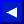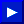### FN

TYPE: Function-Numeric
FORMAT: FN <name> ( <number> )

Action: This function references the previously DEFined formula specified by name. The number is substituted into its place (if any) and the formula is calculated. The result will be a numeric value.

This function can be used in direct mode, as long as the statement DEFining it has been executed.

If an FN is executed before the DEF statement which defines it, an UNDEF'D FUNCTION error occurs.

EXAMPLES of FN (User-Defined) Function:
```   PRINT FN A(Q)
1100 J = FN J(7)+ FN J(9)
9990 IF FN B7 (1+1)= 6 THEN END
```

### FOR ... TO ... [STEP ...]

TYPE: Statement
FORMAT: FOR <variable> = <start> TO <limit> [ STEP <increment> ]

Action: This is a special BASIC statement that lets you easily use a variable as a counter. You must specify certain parameters: the floating-point variable name, its starting value, the limit of the count, and how much to add during each cycle.

Here is a simple BASIC program that counts from 1 to 10, PRINTing each number and ENDing when complete, and using no FOR statements:

```   100 L = 1
110 PRINT L
120 L = 1 + 1
130 IF L <= 10 THEN 110
140 END
```This page has been created by Sami Rautiainen. Read the small print. Last updated December 22, 2002.